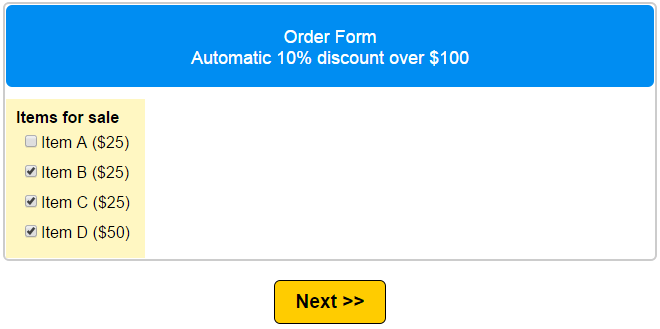# Apply Automatic Discounts for Order Forms

Many online stores have a need for discounts for customers who order over a set dollar amount or quantity. For example:

“All orders over \$100 get 10% off”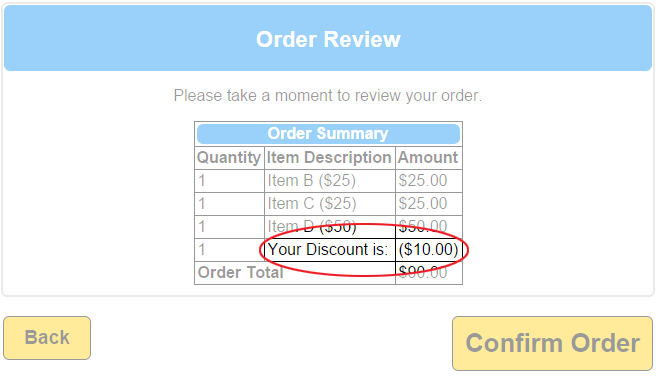Here is how to automate order discounts with your Formsite order form:

Step 1: Start by building your form with all of the order elements you’ll need. In this example, we have 3 items that cost \$25 and 1 item that costs \$50. Next, in the edit window for the order item click on the Calculations tab and enable scoring.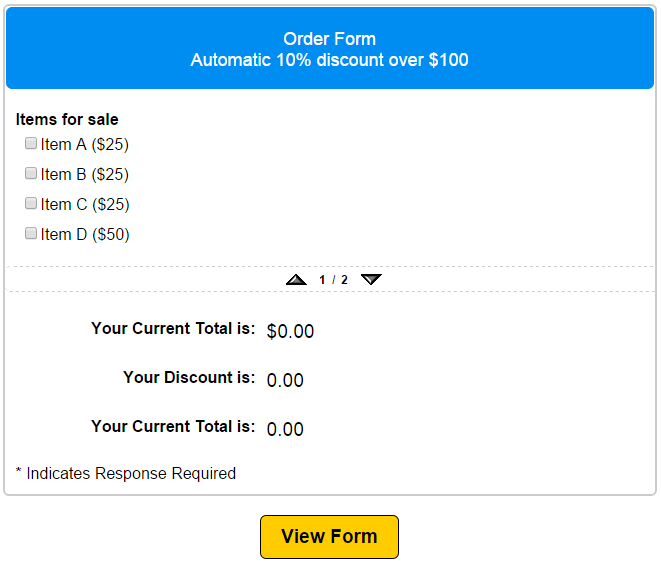Step 2: Next, you want to add your items for both the discount and non-discount order total.

• The non-discount total will be a normal Running Total item.
• The discount elements are 2 Calculation items.

1. Discount calculation: -id1*.1

Start with a negative sign because we’re discounting from the total. Next, the id number of the order item multiplied by our discount (10% = .1).

Note: Be sure to check the box next to Include in Order Total for the discount calculation.

2. Total calculation: id1*.9

This item displays the order total with the discount (1.0 – .1 = .9).

Note: Do not check the box next to Include in Order Total as this item is for display purposes only.

Step 3: Finally, set the Rules to display the appropriate Calculation items based on the form visitor’s selections.

• Non-discount total: Click the Edit link to display the settings window, then click the Rules tab. Set a rule to hide this item when the scoring total is greater than 99. In our case, we are applying the discount for \$100 and over.
• Discount calculation: In the Rules tab, set a rule to hide this item when the scoring total is less than 100.
• Total calculation: Same as discount calculation.

Step 4: Test

If your form visitor selects items that total less than \$100, they see the regular Running Total: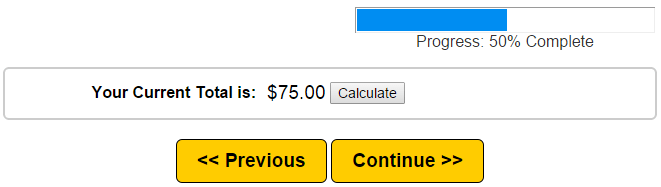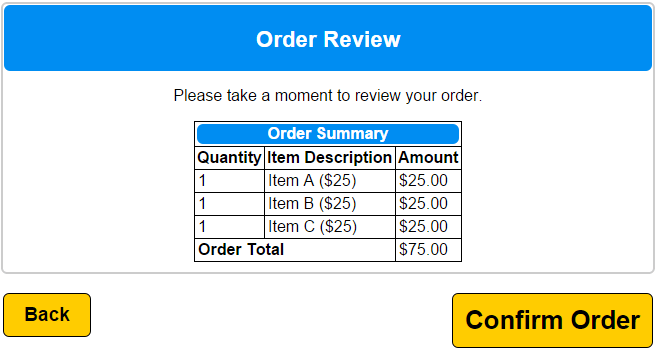If your form visitor selects items that total \$100 or more, they see the Discount calculation and the Discount total: